# may be use proposed circuit. what value should R have? solve (i) and(iii). alpha,beta,gamma,epsilon=1 1. Design...

may be use proposed circuit. what value should R have? solve (i) and(iii). alpha,beta,gamma,epsilon=1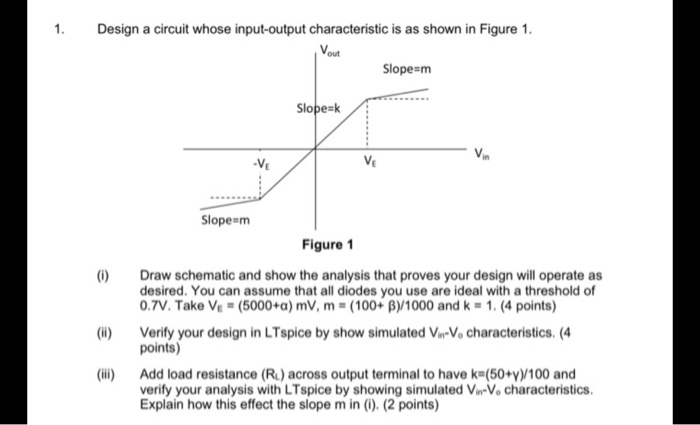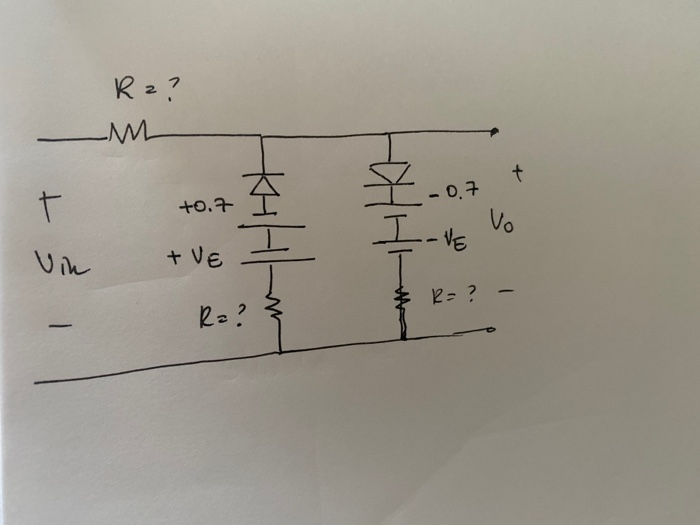1. Design a circuit whose input-output characteristic is as shown in Figure 1. Slope=m Slope=k Slope=m Figure 1 Draw schematic and show the analysis that proves your design will operate as desired. You can assume that all diodes you use are ideal with a threshold of 0.7V. Take Ve- (5000+a) mV, m = (100+ B)/1000 and k = 1. (4 points) Verify your design in LTspice by show simulated V-V. characteristics. (4 points) Add load resistance (R) across output terminal to have k=(50+y)/100 and verify your analysis with LTspice by showing simulated V-V. characteristics Explain how this effect the slope min (0) (2 points)
Rz? -m +0.7 in +VE \$ K-? -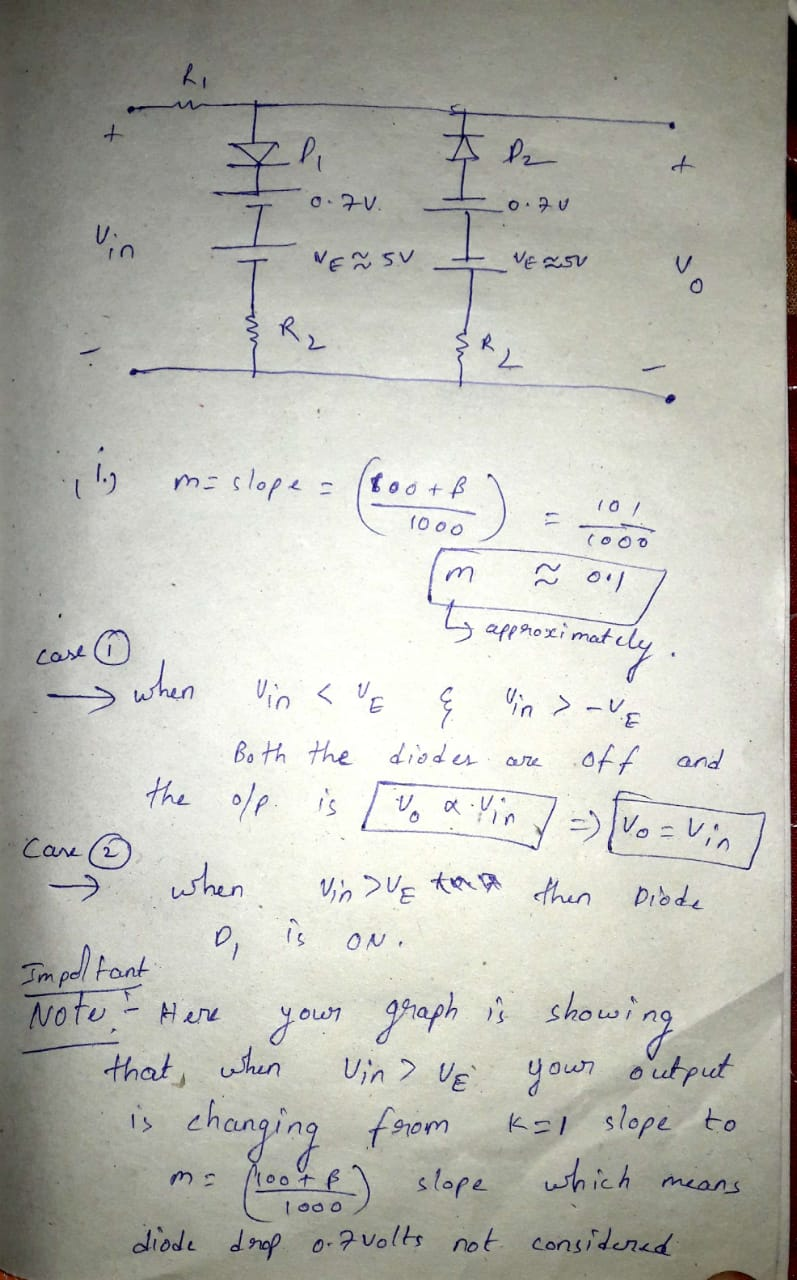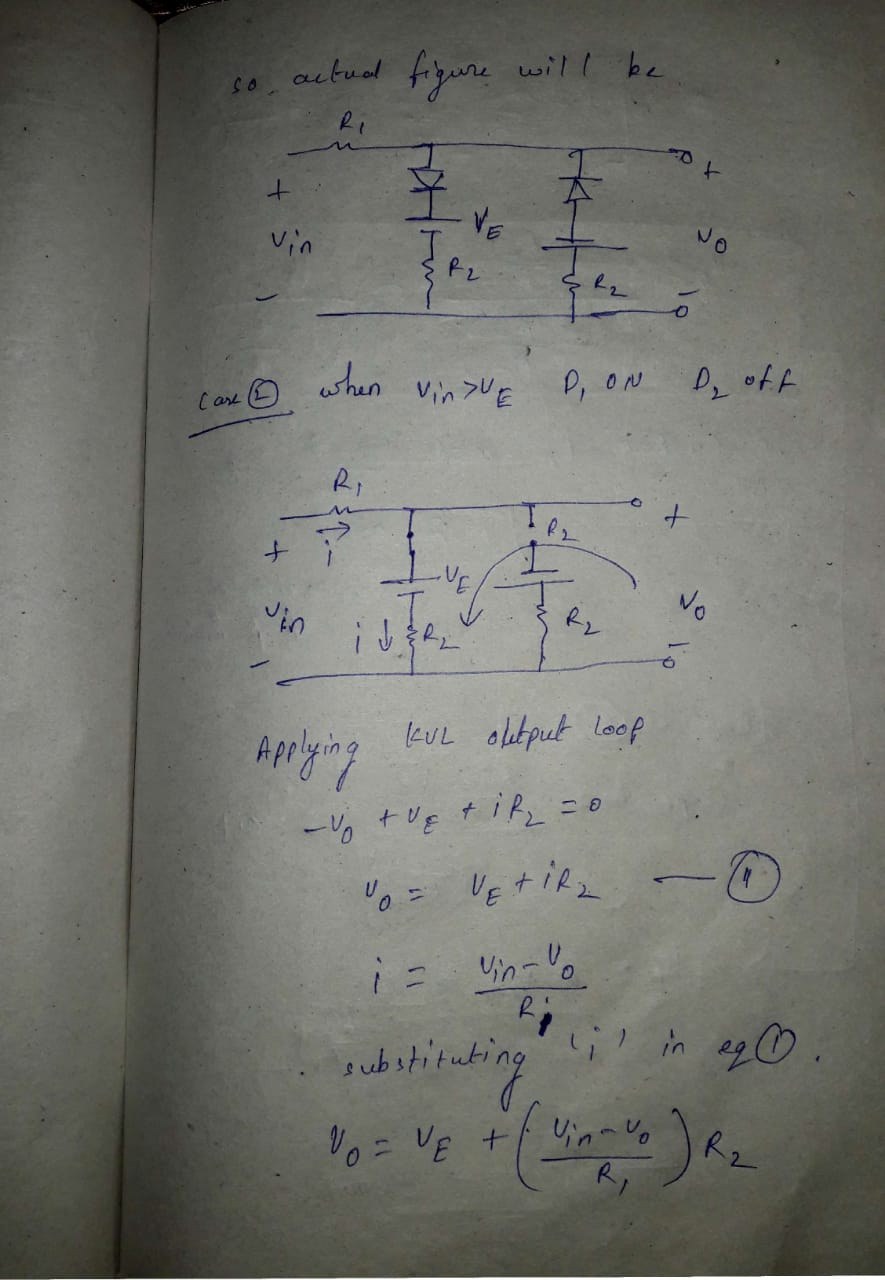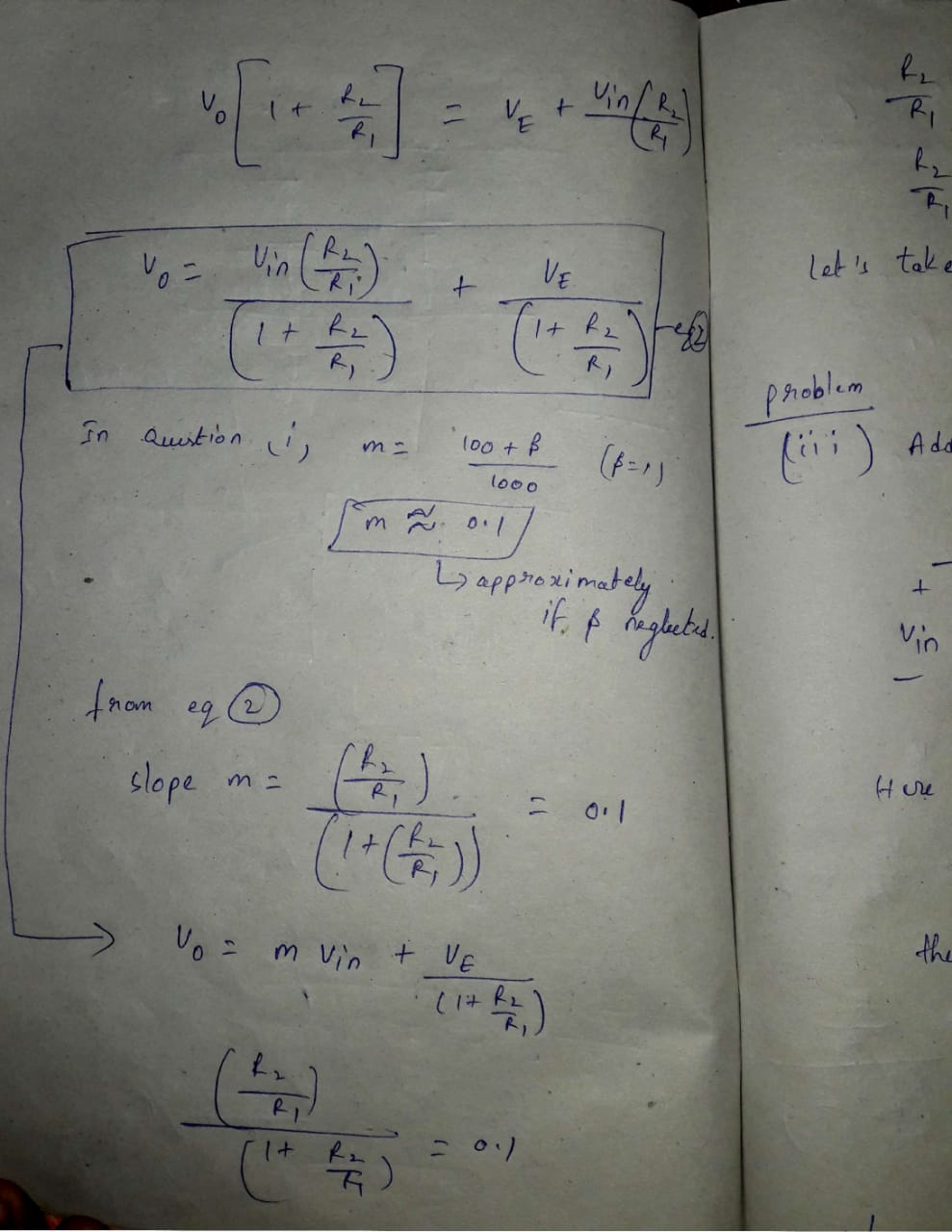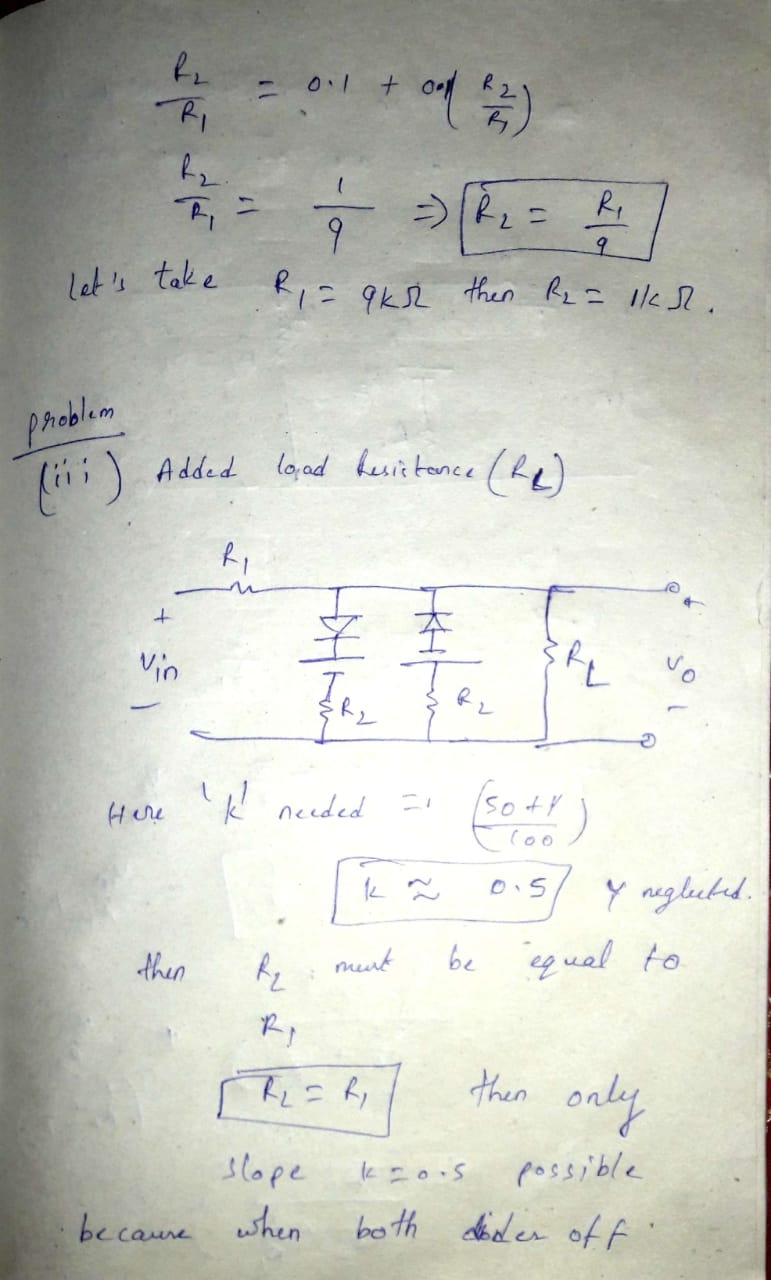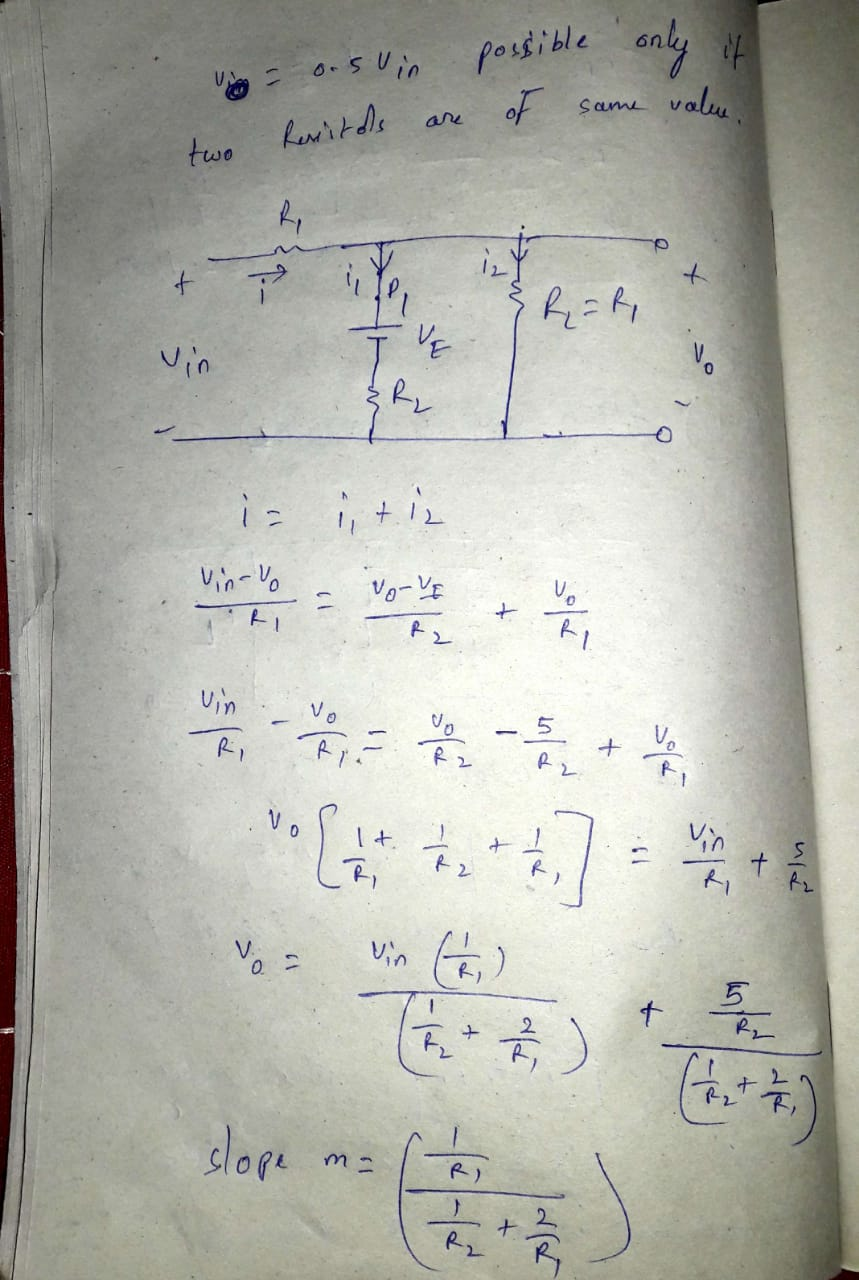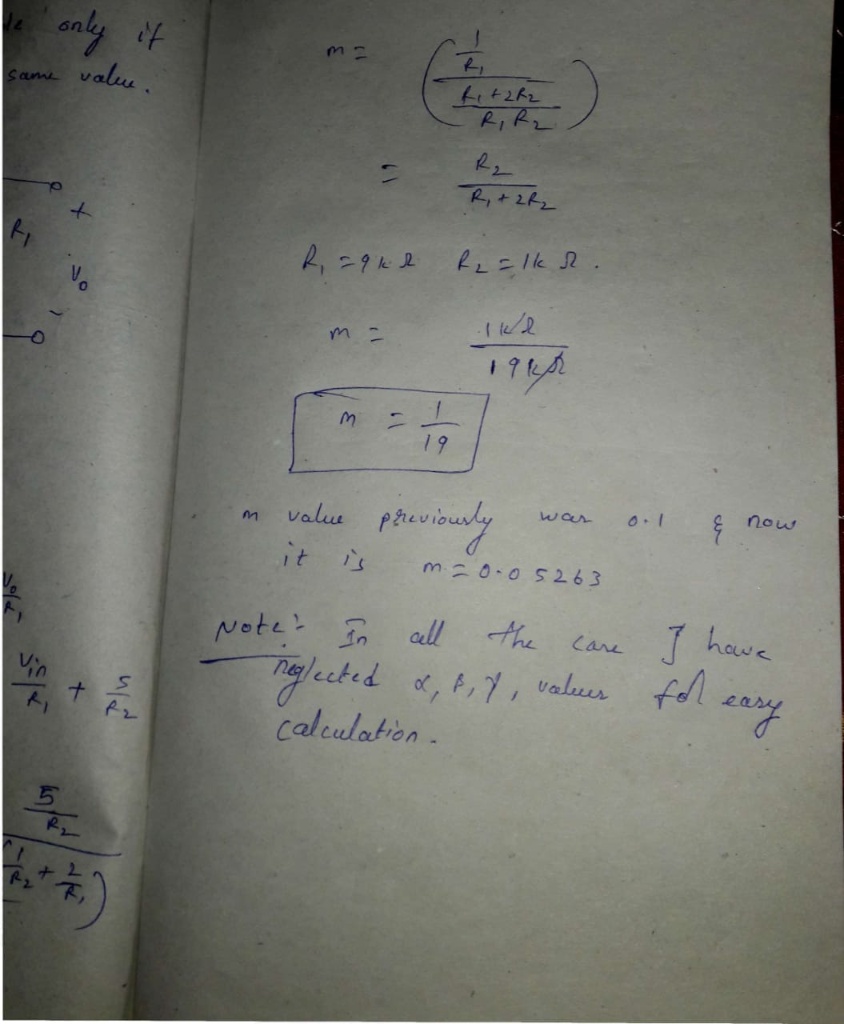##### Add Answer to: may be use proposed circuit. what value should R have? solve (i) and(iii). alpha,beta,gamma,epsilon=1 1. Design...
Similar Homework Help Questions
• ### Do not mind the Greek Alph signs. Please explian steps and write clearly . Many Thanks!...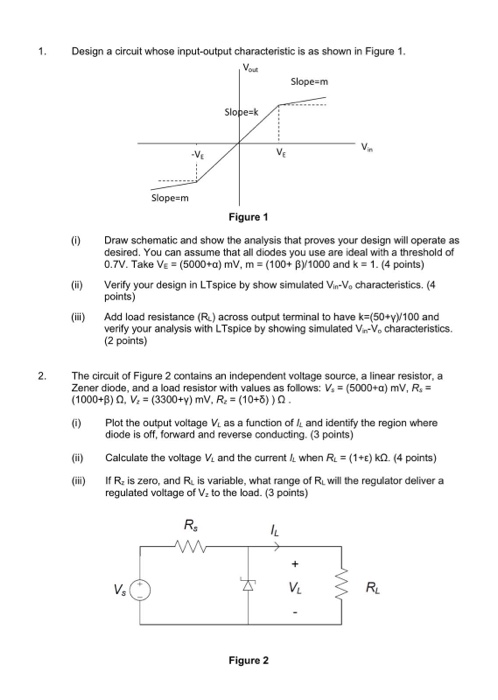Do not mind the Greek Alph signs. Please explian steps and write clearly . Many Thanks! 1. Design a circuit whose input-output characteristic is as shown in Figure 1. Slopem Slopek Ve Slope=m Figure 1 (0) Draw schematic and show the analysis that proves your design will operate as desired. You can assume that all diodes you use are ideal with a threshold of 0.7V. Take Ve = (5000+a) mV, m = (100+ By 1000 and k = 1. (4...

• ### +10V Analysis Problems R voltage OV out 1) Design the given circuit configuration such that the...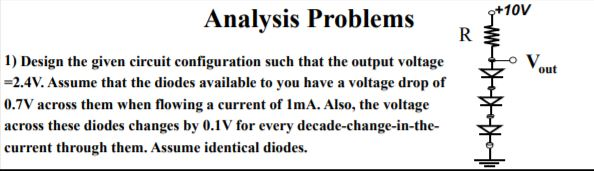+10V Analysis Problems R voltage OV out 1) Design the given circuit configuration such that the output voltage -2.4V. Assume that the diodes available to you have a voltage drop of 0.7V across them when flowing a current of ImA. Also, the voltage across these diodes changes by 0.1V for every decade-change-in-the- current through them. Assume identical diodes. 1

• ### C.la C.1b For the circuit of Figure 1, choose values for resistors R1, R2, and R:...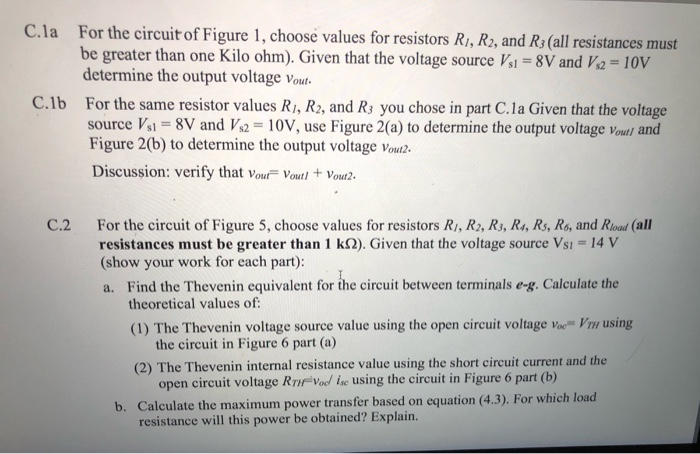C.la C.1b For the circuit of Figure 1, choose values for resistors R1, R2, and R: (all resistances must be greater than one Kilo ohm). Given that the voltage source Vsi = 8V and V2 = 10V determine the output voltage Vout. For the same resistor values R1, R2, and R3 you chose in part C.la Given that the voltage source Vs1 = 8V and Vs2 = 10V, use Figure 2 (a) to determine the output voltage Vout/ and Figure...

• ### please show steps, and solve asap BEE 433 Electronic Circuit Design Problem Set #1 Due date:...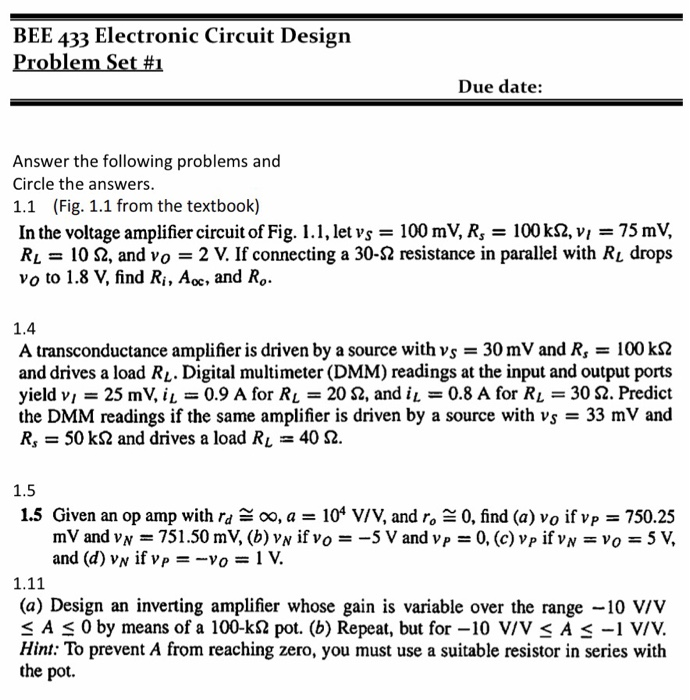please show steps, and solve asap BEE 433 Electronic Circuit Design Problem Set #1 Due date: Answer the following problems and Circle the answers. 1.1 (Fig. 1.1 from the textbook) In the voltage amplifier circuit of Fig. 1.1, let vs = 100 mV, Rs = 100 k 52, Vi = 75 mV, RL = 10 S2, and vo = 2 V. If connecting a 30-S2 resistance in parallel with RL drops vo to 1.8 V, find Ri, A., and R....

• ### I want to find the part1 part2 part3 waveform mathematically. how slove?? please help me!! part 1 part 2 part 3 opampadder ed Q (normal) R3 2k U1 R1 in 1 out V1 1 k R4 100 SINE (0...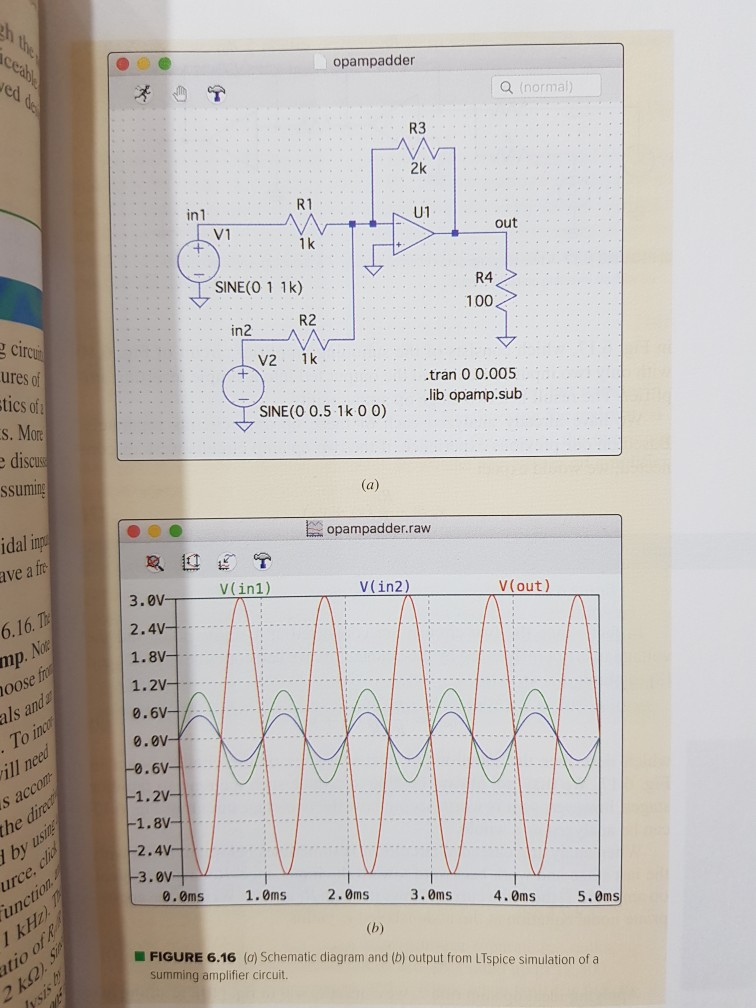I want to find the part1 part2 part3 waveform mathematically. how slove?? please help me!! part 1 part 2 part 3 opampadder ed Q (normal) R3 2k U1 R1 in 1 out V1 1 k R4 100 SINE (0 1 1k) R2 in2 cir ures of tics of s. Mor discu ssuming V2 1k .tran 0 0.005 .lib opamp.sub SINE(0 0.5 1k 0 0) opampadder.raw idal ip ave a fr V(in1) V(in2) V(out) 3.0V 2.4V 1.8V 1.2V 0.6V 0.0V 6.16....

• ### Problem 3: Design Problem On Figure P3a, you have a Common Source (CS) n-channel MOSFET amplifier....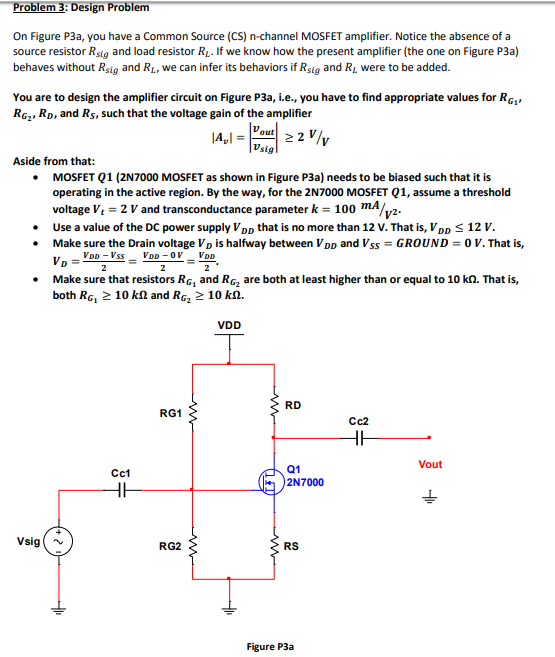Problem 3: Design Problem On Figure P3a, you have a Common Source (CS) n-channel MOSFET amplifier. Notice the absence of a source resistor Rsig and load resistor R. If we know how the present amplifier (the one on Figure P3a) behaves without Rsig and RL, we can infer its behaviors if Rsig and R were to be added. design the amplifier circuit on Figure P3a, i.e., you have to find appropriate values for RGj You are to RG,, RD, and...

• ### I want to solve this problem step by step 1. (35 points) Switch mode DC / DC Converters. a. (15 points) Design a flyback DC/DC power converter to the following specifications. Assume ideal compone...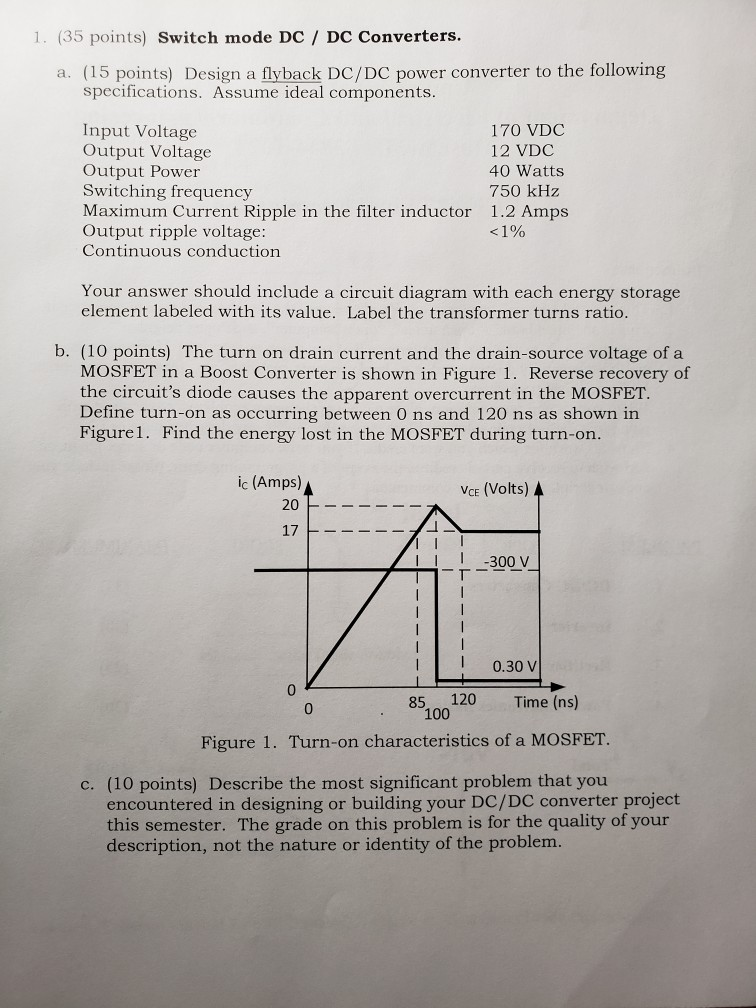I want to solve this problem step by step 1. (35 points) Switch mode DC / DC Converters. a. (15 points) Design a flyback DC/DC power converter to the following specifications. Assume ideal components. Input Voltage Output Voltage Output Power Switching frequency Maximum Current Ripple in the filter inductor Output ripple voltage: Continuous conduction 170 VDC 12 VDC 40 Watts 750 kHz 1.2 Amps Your answer should include a circuit diagram with each energy storage element labeled with its value....

• ### Can I get help with part g through part r, please? Given the following Common-Emitter Amplifier...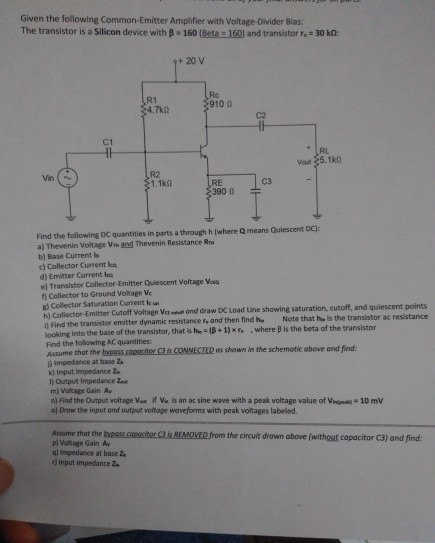Can I get help with part g through part r, please? Given the following Common-Emitter Amplifier with Voltage-Divider Bias: The transistor is a Silicon device with B160 (Beta 160) and transistor r.= 30 kn: 20 V 34.7k LAL = Vou 35.10 31.1k LRE \$3900 03 - Find the following quantities in parts a through where means Quiescent DC): a) Thevenin Voltage and Thevenin Resistance Re b) Base Current la Collector Current le d) Emitter Current le e) Transistor Collector-Emitter Quiescent...

• ### Yes, this is one problem. Please solve ALL PARTS. Guaranteed thumbs up for the person who solves it. 3 1. Photodiode...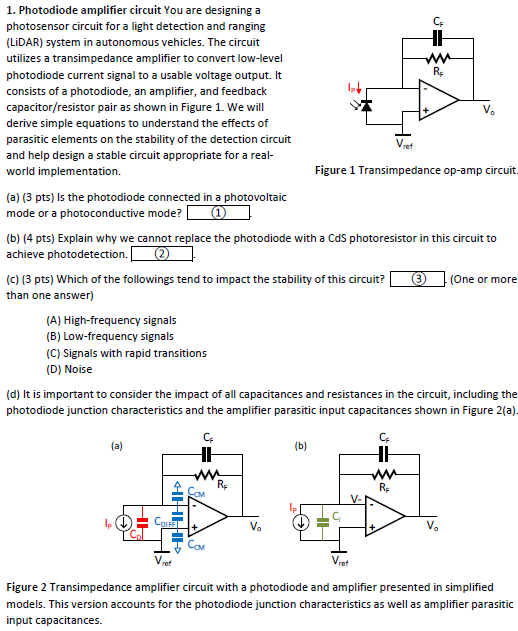Yes, this is one problem. Please solve ALL PARTS. Guaranteed thumbs up for the person who solves it. 3 1. Photodiode amplifier circuit You are designinga CF photosensor circuit for a light detection and ranging LiDAR) system in autonomous vehicles. The circuit utilizes a transimpedance amplifier to convert low-level RF photodiode current signal to a usable voltage output. It consists of a photodiode, an amplifier, and feedback capacitor/resistor pair as shown in Figure 1. We will derive simple equations to...

• ### I am currently trying to figure out the experiment below. Please complete Table 1 with an...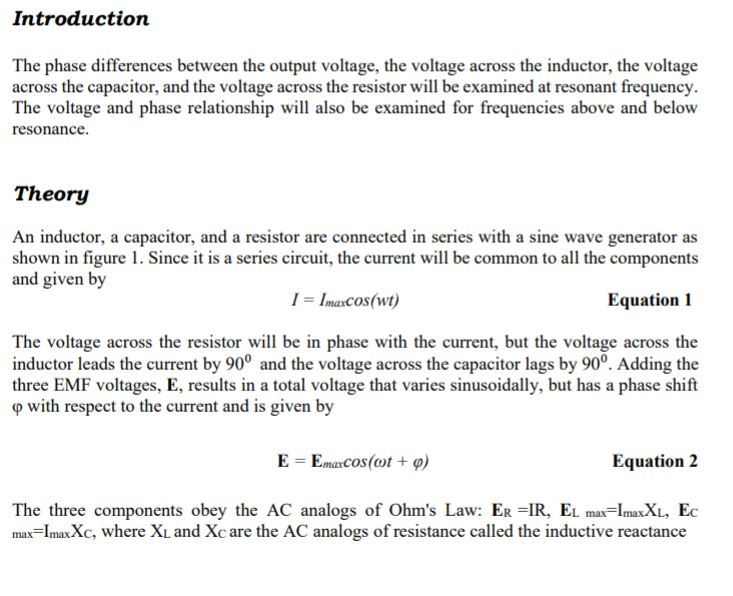I am currently trying to figure out the experiment below. Please complete Table 1 with an explanation, I appreciate it thank you!  Promise to give thumbs up! Introduction The phase differences between the output voltage, the voltage across the inductor, the voltage across the capacitor, and the voltage across the resistor will be examined at resonant frequency. The voltage and phase relationship will also be examined for frequencies above and below resonance. Theory An inductor, a capacitor, and a resistor are...

Free Homework App